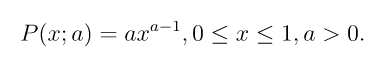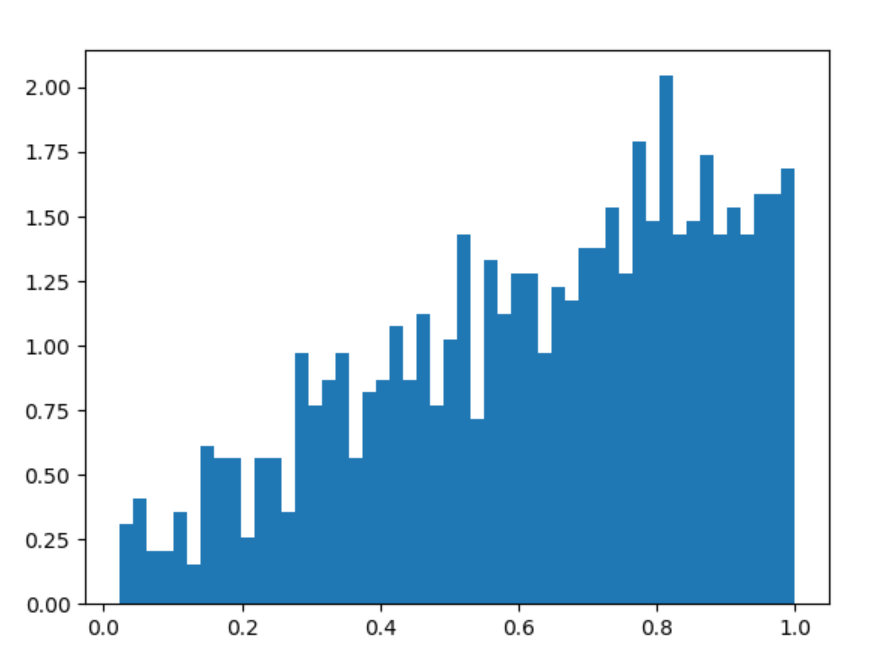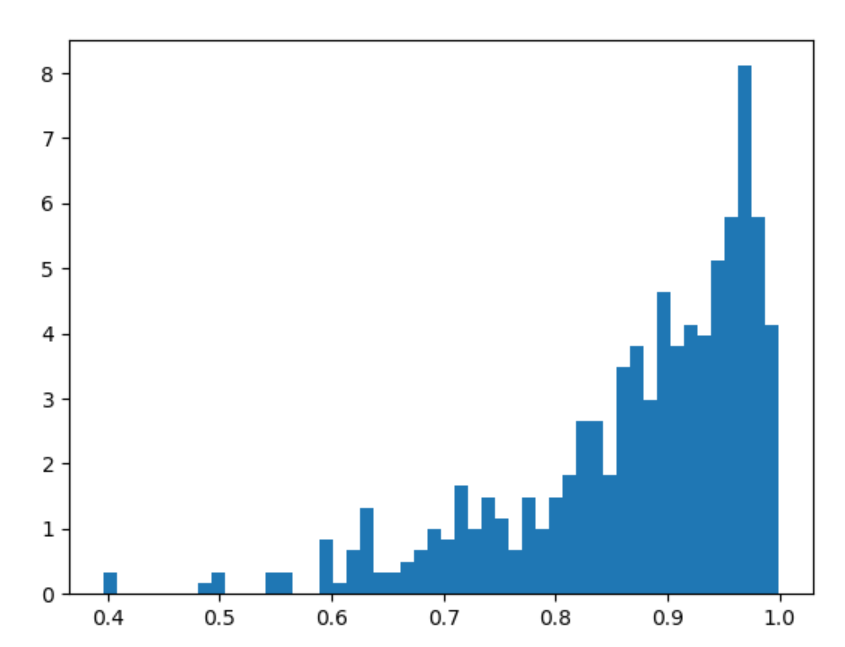# numpy.random.power() in Python

• Last Updated : 15 Jul, 2020

With the help of numpy.random.power() method, we can get the random samples from power distribution and return the random samples by using this method.power distribution

Syntax : numpy.random.power(a, size=None)

Return : Return the random samples as numpy array.

Example #1 :

In this example we can see that by using numpy.random.power() method, we are able to get the random samples from power distribution and return the random samples.

## Python3

 `# import numpy``import` `numpy as np``import` `matplotlib.pyplot as plt`` ` `# Using power() method``gfg ``=` `np.random.power(``1.75``, ``1000``)`` ` `plt.figure()``plt.hist(gfg, bins ``=` `50``, density ``=` `True``)``plt.show()`

Output :power plot

Example #2 :

## Python3

 `# import numpy``import` `numpy as np``import` `matplotlib.pyplot as plt`` ` `# Using power() method``gfg ``=` `np.random.power(``6.75``, ``500``)`` ` `plt.figure()``plt.hist(gfg, bins ``=` `50``, density ``=` `True``)``plt.show()`

Output :power plot

My Personal Notes arrow_drop_up